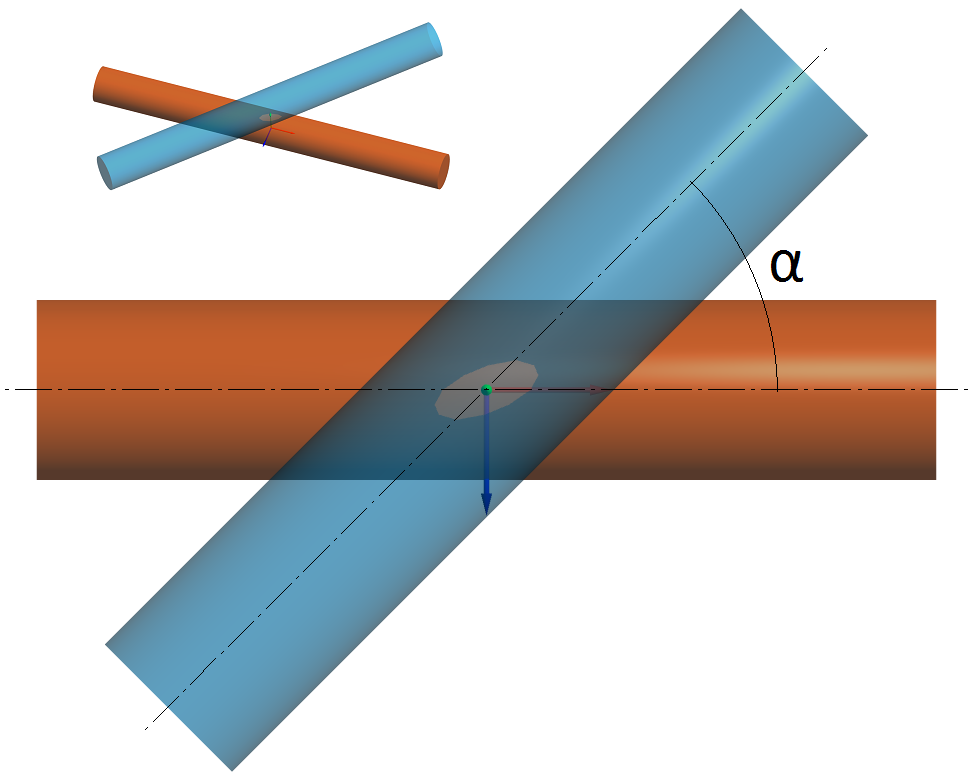﻿ Angle between axes

# MESYS Calculation Software

 Navigation: MESYS Hertz Calculation > Input Parameters > General Angle between axes

The radii of curvature for two bodies can be given in two planes and an angle between these planes can be specified. The angle is defined between the lines which are normal to the planes where the radii r11 and r21 lie, as shown in the picture: